Altair

# Altair Tutorial¶

This tutorial presents an overview of the Altair plotting module in Python. Altair is a powerful tool for creating professional-looking plots that is also extremely customizable. Rather than providing a complete overview, we focus on basic features specifically relevant to exploratory data analysis. Altair directly works on Pandas data frames. The key idea in Altair is that we declare the links between data columns and visual encoding channels. The rest of the plotting details are handled automatically by Altair. And of course, we can always change the default behavior. Altair's "grammar of graphics" logic is a natural extension of how we think about data and it is similar to that of ggplot2 in R, making Altair an attractive option for plotting in Python. However, an advantage of Altair over ggplot2 is that Altair allows for interactive charts, including zooming, panning, and tooltips. Installation details of Altair can be found here. This tutorial requires Altair version 3.0 or above (which requires Jupyter Lab version 1.0 or above). Admittedly, some of the plots in this tutorial could be more pretty, but our focus here is on functionality rather than visual perfection.

We use the diamonds dataset from the ggplot2 library in R in our examples. Let's load the diamonds data from the Cloud as a csv file into a Pandas data frame.
In :
%config InlineBackend.figure_format = 'retina'

import altair as alt
alt.renderers.enable('notebook')

import warnings
warnings.filterwarnings("ignore")

import numpy as np
import pandas as pd
import io
import requests
import os, ssl

if (not os.environ.get('PYTHONHTTPSVERIFY', '') and
getattr(ssl, '_create_unverified_context', None)):
ssl._create_default_https_context = ssl._create_unverified_context

diamonds_url = 'https://raw.githubusercontent.com/vaksakalli/datasets/master/diamonds.csv'
url_content = requests.get(diamonds_url).content
df_full.shape

Out:
(53940, 10)
The diamonds dataset has more than 50K rows, so let's get a random subset with 500 observations and have a look at 5 randomly selected rows.
In :
df = df_full.sample(n=500, random_state=8)
df.sample(n=5, random_state=8)

Out:
carat cut color clarity depth table x y z price
15714 0.36 Premium G SI2 59.3 59.0 4.66 4.62 2.75 608
978 0.81 Ideal G SI2 62.2 57.0 5.96 6.00 3.72 2894
24334 2.02 Very Good J SI1 59.8 59.9 8.16 8.21 4.90 12598
16282 1.53 Premium H SI2 61.5 60.0 7.46 7.39 4.56 6512
27876 0.40 Ideal G SI2 61.8 56.0 4.75 4.77 2.94 654
As part of exploratory analysis, let's get the categorical columns and print their unique value counts.
In :
[df[cat_col].value_counts()
for cat_col in df.columns[df.dtypes==object].tolist()]

Out:
[Ideal        202
Very Good    106
Good          48
Fair          15
Name: cut, dtype: int64, G    93
E    91
F    89
H    88
D    64
I    52
J    23
Name: color, dtype: int64, VS2     126
SI1     111
SI2      87
VS1      77
VVS2     37
VVS1     35
IF       19
I1        8
Name: clarity, dtype: int64]
Let's also get a summary of the numerical columns.
In :
df.describe().round(3)

Out:
carat depth table x y z price
count 500.000 500.000 500.000 500.000 500.000 500.000 500.000
mean 0.790 61.717 57.444 5.708 5.711 3.524 3845.190
std 0.488 1.406 2.167 1.127 1.122 0.697 3978.464
min 0.230 55.200 52.000 3.880 3.860 2.370 394.000
25% 0.400 61.000 56.000 4.720 4.738 2.900 930.250
50% 0.700 61.800 57.000 5.625 5.630 3.490 2200.000
75% 1.020 62.500 59.000 6.490 6.492 4.012 5144.000
max 3.650 67.100 65.000 9.530 9.480 6.380 18757.000

## Altair Basics¶

### Charts¶

Altair works on Pandas data frames. The primary object in Altair is the Chart object that takes a data frame as a single input argument.

### Marks¶

Once a chart object is defined, next step is to specify the "mark". Marks are fundamental in Altair as they specify how we want our data to be visualized. The mark attribute of a chart object is specified via the Chart.mark_* methods. Some common mark types are as follows:
• mark_bar(): A bar plot such as a histogram
• mark_line(): A line plot
• mark_point(): A scatter plot whose points can be configured as desired
• mark_boxplot(): A boxplot
You can customize Altair marks using their "property channels", such as color, fill, opacity, size, and shape.

### Encodings¶

Subsequent to specifying a mark, we define the "encoding" of the visualization channels, which are practically the $x$ and $y$ axes (sometimes together with a color parameter for 3-variable plots). A unique Altair feature is that you can tell Altair how you want a particular data frame column to be treated. Altair defaults to "quantitative" for numeric data, "temporal" for date and time data, and "nominal" for string data. However, you can force Altair to treat a numerical column as "nominal", for instance. You can also tell Altair that a particular column is of "ordinal" type. Thus, if you are not happy with how Altair treats your columns, you can override them using the encoding data types below:
• Quantitative (Q): A real-valued quantity
• Ordinal (O): An ordinal categorical quantity
• Nominal (N): A nominal categorical quantity
• Temporal (T): A time or date quantity
The syntax for specifying encoding data types is "column:Type".

### Data Aggregation¶

For flexibility and convenience, Altair has a built-in capability for data aggregation such as averaging, counts, etc.

## Bar Charts¶

Let's first import the Altair module. The common convention is to import Altair as "alt". Next, let's do the following:
• Define a Chart object with the diamonds dataset,
• Set the mark to "bar", and
• Encode $x$ and $y$ channels as "cut" and "count()" respectively for a bar chart for the "cut" column.
In :
alt.Chart(df).mark_bar().encode(x='cut', y='count()')

Out: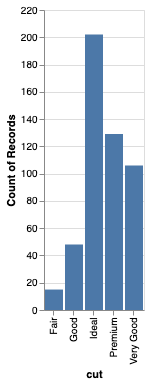Let's now do something fancy. Let's add the clarity dimension to the above plot the by setting color to "clarity".
In :
alt.Chart(df).mark_bar().encode(
x='cut',
y='count()',
color='clarity')

Out: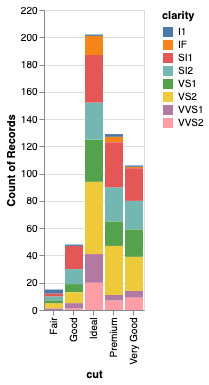Let's see how the plot above changes when we set the data type of "clarity" column to ordinal. Of course, the levels of this column is sorted alphabetically by default, which is probably not correct. So, in reality, we would have to supply the correct ordering of levels for sorting.
In :
alt.Chart(df).mark_bar().encode(
x='cut',
y='count()',
color='clarity:O')

Out: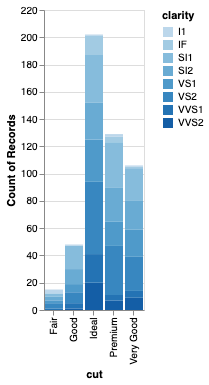This plot is somewhat dry. So, let's customize it a bit. For customization, we need to be more specific with the $x$ and $y$ axes and also the color parameter by using the X, Y, and Color methods in Altair so that we can supply some additional parameters. Below, we pass in a list for the correct ordering of "cut", which is ordinal. Otherwise, the sorting would be done alphabetically. If you want to skip sorting altogether, you need to say "sort=None". By default, if an $x$ axis column is categorical, its labels are printed vertically. To change this, we need to specify a label angle as below. We also set the plot width to 500 pixels and add labels to the axes to together with a plot title. These embellishments can be applied to other charts as well.
In :
alt.Chart(df,
width=500,
title='Cut Distribution'
).mark_bar(opacity=0.9,
color='blue'
).encode(x=alt.X('cut',
title='Cut Type',
sort=['Fair', 'Good', 'Very Good', 'Premium', 'Ideal'],
axis=alt.AxisConfig(labelAngle=45)),
y=alt.Y('count()', title='Observation Count'),
color=alt.Color('clarity')
)

Out: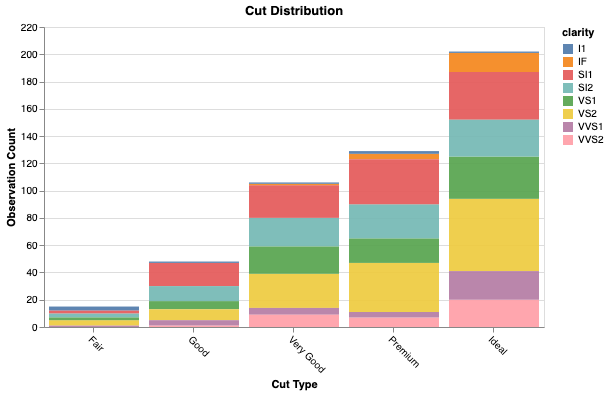## Histograms¶

Histograms can be plotted with a "bar" mark and we can specify the maximum number of bins we want in our histogram.
In :
alt.Chart(df).mark_bar(color='green').encode(
alt.X('carat', bin=alt.Bin(maxbins=20)),
y='count()')

Out: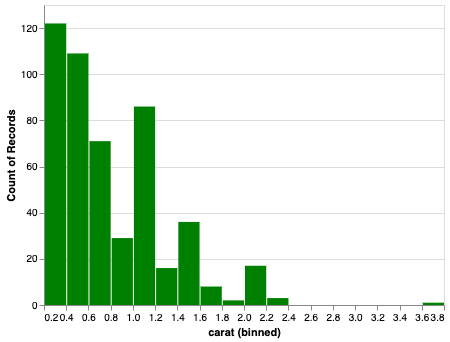We can also specify a "color" parameter for a histogram as below.
In :
alt.Chart(df).mark_bar().encode(
alt.X('carat', bin=alt.Bin(maxbins=20)),
y='count()',
color='cut')

Out: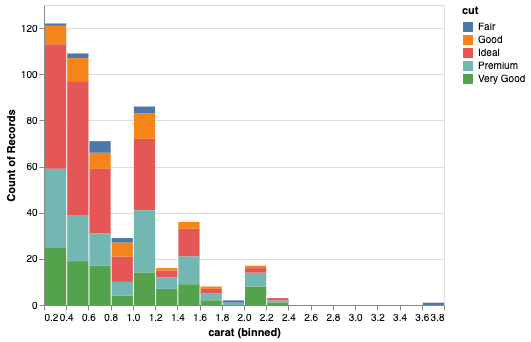## Boxplots¶

Boxplots are plotted with a "boxplot" mark. Let's display a boxplot for the "carat" column with the full range of values.
In :
alt.Chart(df).mark_boxplot(extent='min-max').encode(x='carat')

Out: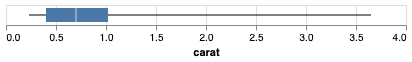Next, let's use the default value of 1.5 IQR for the "extent" parameter. In this version, outlier values are shown as circles.
In :
alt.Chart(df).mark_boxplot().encode(x='carat')

Out: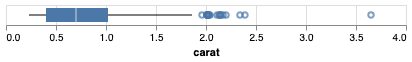We can display multiple boxplots with respect to a categorical column. Below we switch the axes for a vertical display.
In :
alt.Chart(df, width=300).mark_boxplot().encode(y='carat', x='cut')